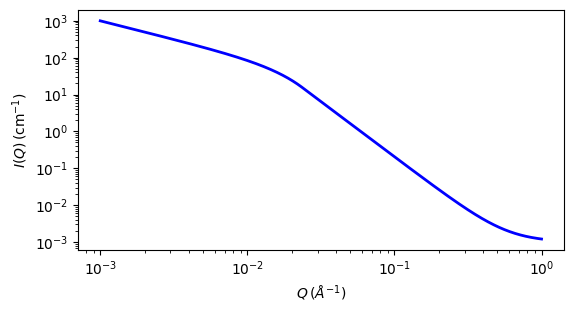# guinier_porod

Guinier-Porod function

Parameter

Description

Units

Default value

scale

Scale factor or Volume fraction

None

1

background

Source background

cm-1

0.001

rg

60

s

Dimension variable

None

1

porod_exp

Porod exponent

None

3

The returned value is scaled to units of cm-1 sr-1, absolute scale.

Calculates the scattering for a generalized Guinier/power law object. This is an empirical model that can be used to determine the size and dimensionality of scattering objects, including asymmetric objects such as rods or platelets, and shapes intermediate between spheres and rods or between rods and platelets, and overcomes some of the deficiencies of the (Beaucage) unified_power_Rg model (see Hammouda, 2010).

Definition

The following functional form is used

$\begin{split}I(q) = \begin{cases} \frac{G}{Q^s}\ \exp{\left[\frac{-Q^2R_g^2}{3-s} \right]} & Q \leq Q_1 \\ D / Q^m & Q \geq Q_1 \end{cases}\end{split}$

This is based on the generalized Guinier law for such elongated objects (see the Glatter reference below). For 3D globular objects (such as spheres), $$s = 0$$ and one recovers the standard Guinier formula. For 2D symmetry (such as for rods) $$s = 1$$, and for 1D symmetry (such as for lamellae or platelets) $$s = 2$$. A dimensionality parameter ($$3-s$$) is thus defined, and is 3 for spherical objects, 2 for rods, and 1 for plates.

Enforcing the continuity of the Guinier and Porod functions and their derivatives yields

$Q_1 = \frac{1}{R_g} \sqrt{(m-s)(3-s)/2}$

and

\begin{align}\begin{aligned}D &= G \ \exp{ \left[ \frac{-Q_1^2 R_g^2}{3-s} \right]} \ Q_1^{m-s}\\ &= \frac{G}{R_g^{m-s}} \ \exp \left[ -\frac{m-s}{2} \right] \left( \frac{(m-s)(3-s)}{2} \right)^{\frac{m-s}{2}}\end{aligned}\end{align}

Note that the radius of gyration for a sphere of radius $$R$$ is given by $$R_g = R \sqrt{3/5}$$. For a cylinder of radius $$R$$ and length $$L$$, $$R_g^2 = \frac{L^2}{12} + \frac{R^2}{2}$$ from which the cross-sectional radius of gyration for a randomly oriented thin cylinder is $$R_g = R/\sqrt{2}$$ and the cross-sectional radius of gyration of a randomly oriented lamella of thickness $$T$$ is given by $$R_g = T / \sqrt{12}$$.

For 2D data: The 2D scattering intensity is calculated in the same way as 1D, where the q vector is defined as

$q = \sqrt{q_x^2+q_y^2}$Fig. 107 1D plot corresponding to the default parameters of the model.

Source

guinier_porod.py

Reference

1. B Hammouda, A new Guinier-Porod model, J. Appl. Cryst., (2010), 43, 716-719

2. B Hammouda, Analysis of the Beaucage model, J. Appl. Cryst., (2010), 43, 1474-1478

Authorship and Verification

• Author: